Tamilnadu State Board New Syllabus Samacheer Kalvi 12th Business Maths Guide Pdf Chapter 10 Operations Research Miscellaneous Problems Text Book Back Questions and Answers, Notes.

## Tamilnadu Samacheer Kalvi 12th Business Maths Solutions Chapter 10 Operations Research Miscellaneous Problems

Question 1.
The following table summarizes the supply, demand and cost information for four factors S1, S2, S3, S4 Shipping goods to three warehouses D1, D2, D3.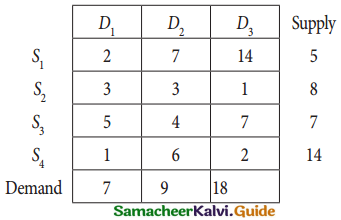Find an initial solution by using north west corner rule. What is the total cost for this solution?
Solution:
The given transportation table is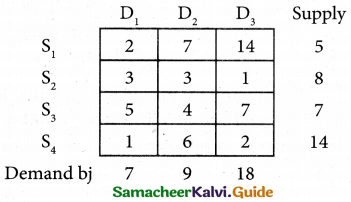Here total supply = 5 + 8 + 7+14 = 34
Total amount =7 + 9 + 18 = 34
(i.e) Total supply = Total demand.
∴ The given problem is balanced transformation problem.
We can find an initial basic feasible solution to the given problem.
First allocation: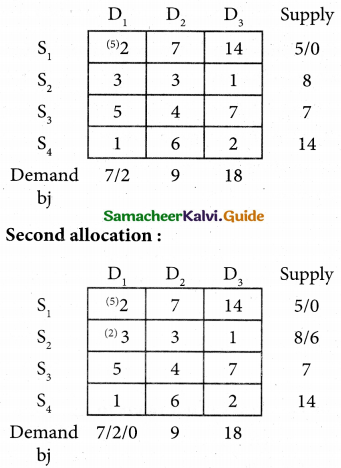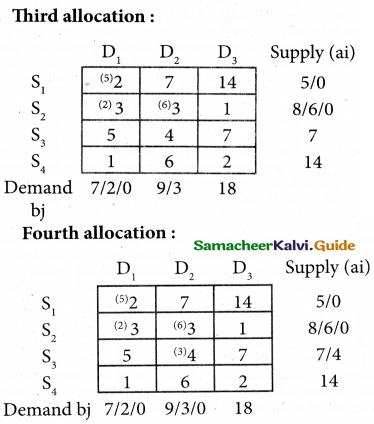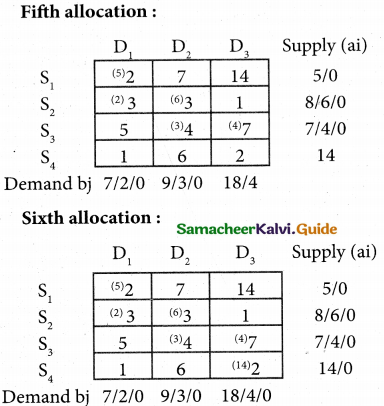Transportation schedule:
S1 → D1; S2 → D1; S2 → D2;
S3 → D2; S3 → D3; S4 → D3
The transportation cost:
= (5 × 2) + (2 × 3) + (6 × 3) + (3 × 4) + (4 × 7) + (14 × 2)
= 10 + 6 + 18 + 12 + 28 + 28
= 102Question 2.
Consider the following transportation problem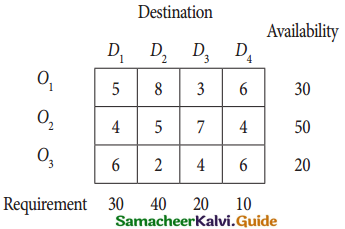Determine an initial basic feasible solution using (a) Least cost method (b) Vogel’s approximation method.
Solution:
(a) Least cost method
Given transportation table is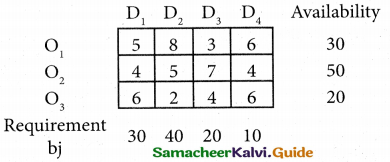Total Availability = Total Requirement = 100
∴ The given problem is balanced transformation problem.
Hence there exists a feasible solution to the given problem
First allocation: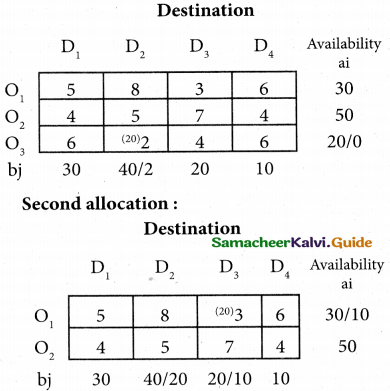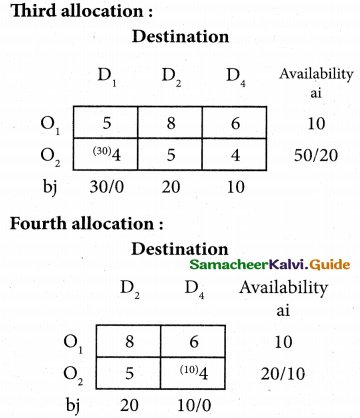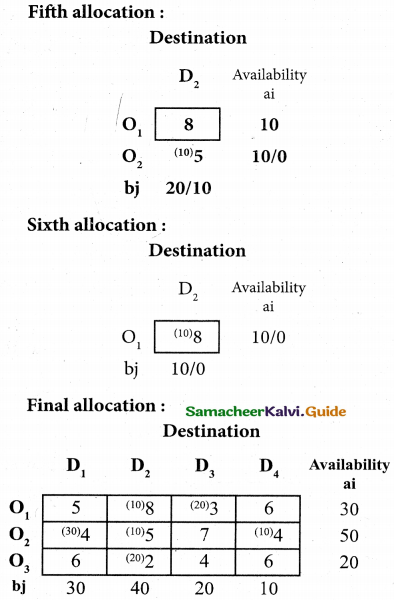Here
x12 = 10; x13 = 20; x21 = 30;
x22 = 10; x24 = 10; x32 = 20
Transportation Scheme:
O1 → D2; O1 → D3; O2 → D1;
O2 → D2; O2 → D4; O3 → D2
The transportation cost:
=(10 × 8) + (20 × 3) + (30 × 4) + (10 × 5) + (10 × 4) + (20 × 2)
= 80 + 60 + 120 + 50 + 40 + 40
= 390

(ii) Vogel’s approximation method:
Here Σai = Σbj = 100
∴ The given problem is balanced transformation problem.
Hence there exists a feasible solution to the given problem
First allocation: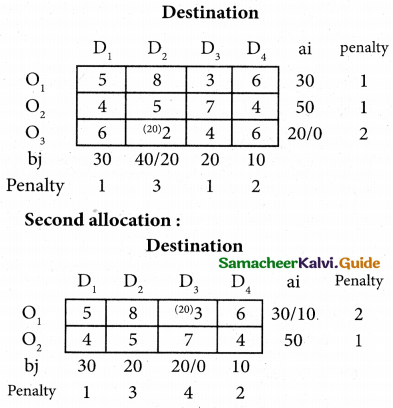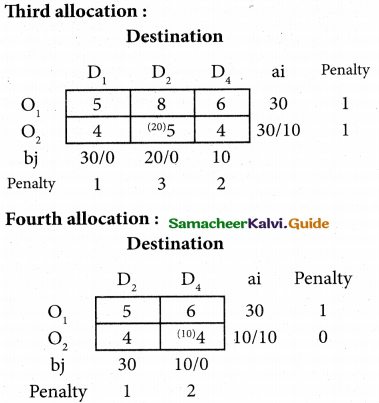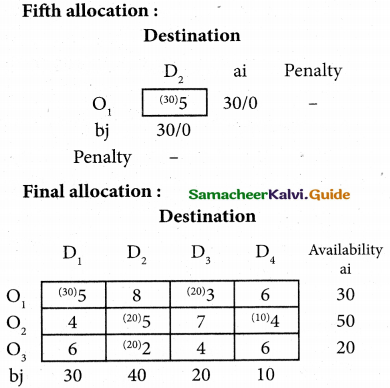Here
x11 = 30; x13 = 20; x22 = 20;
x24 = 10; x32 = 20
Transportation Scheme:
O1 → D1; O2 → D3; O2 → D2;
O2 → D4; O3 → D2;
Total transportation cost:
= (30 × 5) + (20 × 3) + (20 × 5) + (10 × 4) + (20 × 2)
= 150 + 60 + 100 + 40 + 40
= 390Question 3.
Determine an initital basic feasible solution to the following transportation problem by using (i) North West Corner rule (ii) least cost method.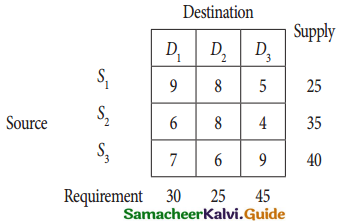Solution:
(i) North West Corner rule:
The given transportation table is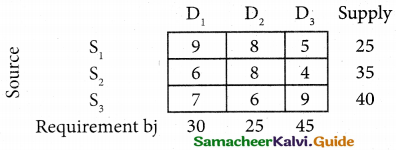Total supply = 25 + 35 + 40 = 100
Total Requirement = 30 + 25 + 45 + 100
(i.e) Total supply = Total requirement.
∴ The given problem is balanced transformation problem.
Hence there exists a feasible solution to the given problem
First allocation: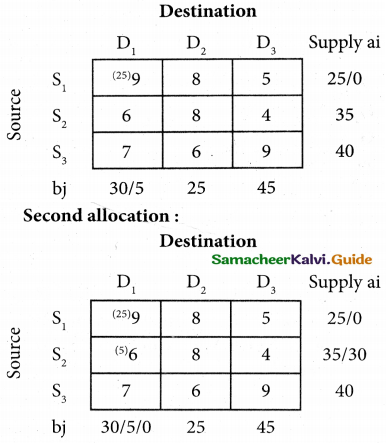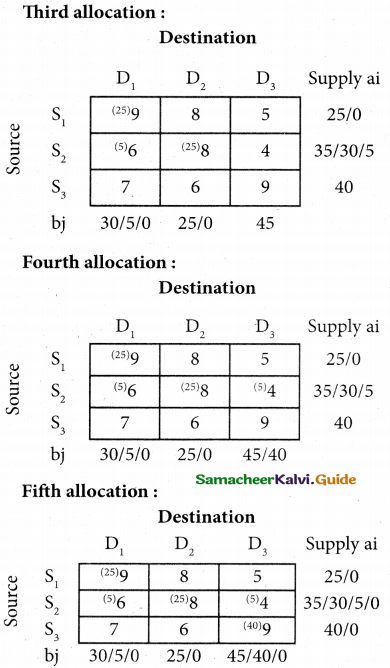Transformation schedule:
S1 → D1; S2 → D1; S2 → D2;
S3 → D3; S3 → D3;
The transformation cost:
= (25 × 9) + (5 × 6) + (25 × 8) + (5 × 4) + (40 × 9)
= 225 + 30 + 200 + 20 + 360 = 835

(ii) Least cost method
The given transportation table is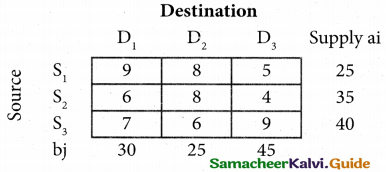(i.e) Total supply = Total requirement = 100
∴ The given problem is balanced transformation problem.
Hence there exists a feasible solution to the given problem
First allocation: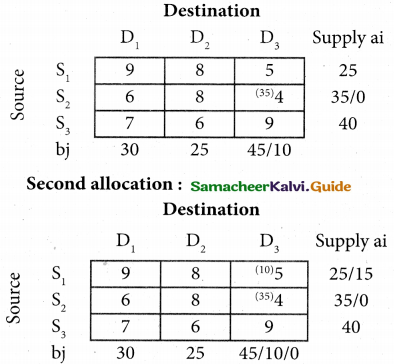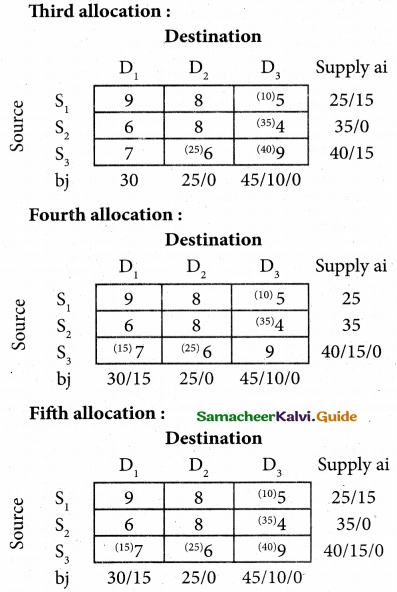Transportation schedule:
S1 → D3; S2 → D3; S3 → D1
S3 → D2;
The transportation cost:
= (10 × 5) + (35 × 4) + (15 × 7) + (25 × 6) + (40 × 9)
= 50 + 140 + 105 + 150 + 360 = 805Question 4.
Explain Vogel’s approximation method by obtaining initial basic feasible solution of the following transportation problem.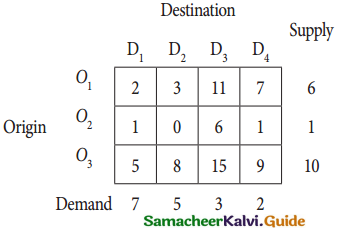Solution:
The given transportation table is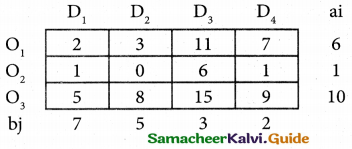Here Σai = Σbj =17
(i.e) Total supply = Total Demand
∴ The given problem is balanced transformation problem.
Hence there exists a feasible solution to the given problem
First allocation: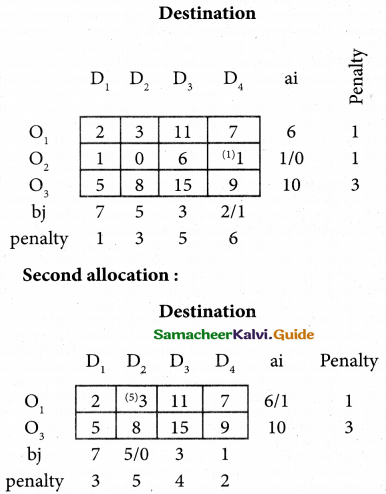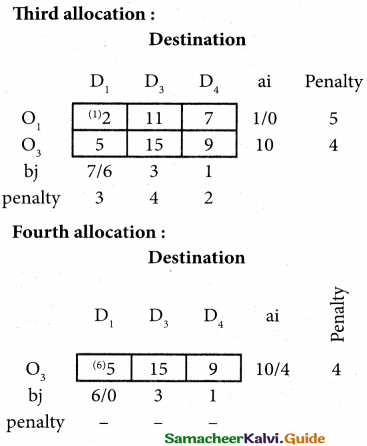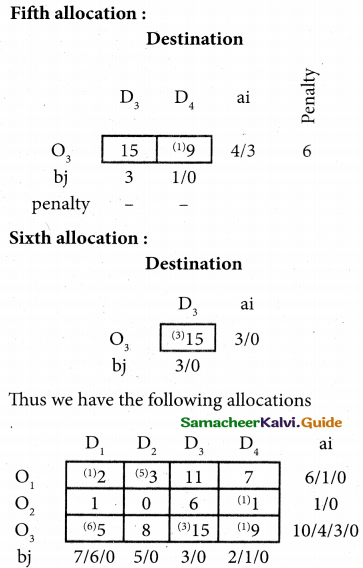Here
x11 = 1; x12 = 5; x24 = 1;
x31 = 6; x33 = 15;
Transportation schedule:
O1 → D1; O1 → D2; O2 → D4
O3 → D1; O3 → D3
The transportation cost:
=(1 × 2) + (5 × 3) + (1 × 1) + (6 × 5) + (3 × 15) + (1 × 9)
= 2 + 15 + 1 + 30 + 45 + 9
= 102Question 5.
A car hire company has one car at each of five depots a,b,c,d and e. A customer in each of the fine towers A, B, C, D and E requires a car. The distance (in miles) between the depots (origins) and the towers (destinations) where the customers are given the following distance matrix.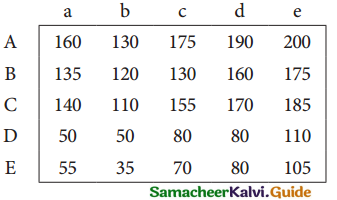How should the cars be assigned to the customers so as to minimize the distance travelled?
Solution:
Here the number of rows and columns are equal.
∴ The given assignment problem is balanced.
Step 1.
Select the smallest element in each row and subtract this from all the elements in its row.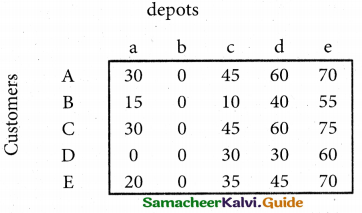Step 2.
Select the smallest element in each column and subtract this from all the elements in its column.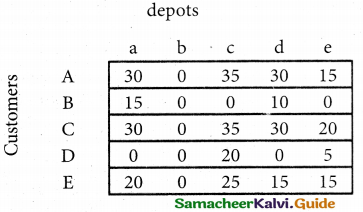Step 3. (Assignment)
Examine the rows with exactly one zero, mark the zero by □ mark other zeros, in its column by X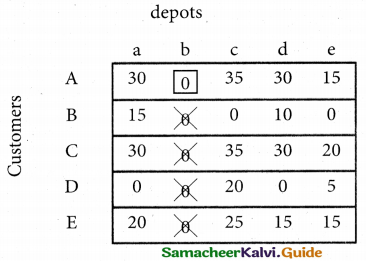Step 4.
Now Examine the rows with exactly one zero, mark the zero by □ mark other zeros, in its column by X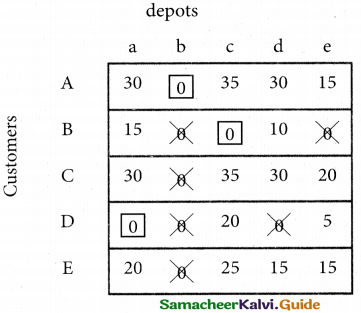Step 5.
Cover all the zeros of table 4 with three lives. Since three assignments were made please note that check [✓] Row C and E which have no assignment.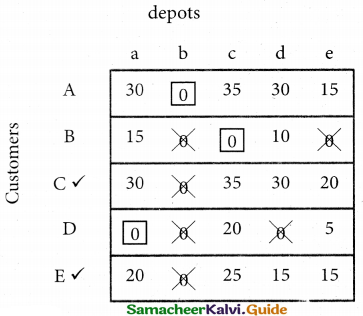Step 6.
Develop the new revised tableau. Examine those elements that are not covered by a line in Table 5. Take the smallest element in each row and subtract from the uncovered cells, depots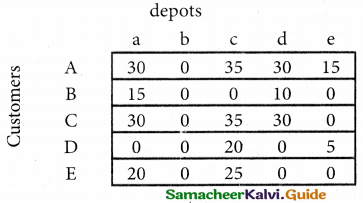Step 7.
Go to step 3 and repeat the procedure until you arrive at an optimal assignments.
depots

Step 8.
Determine an assignment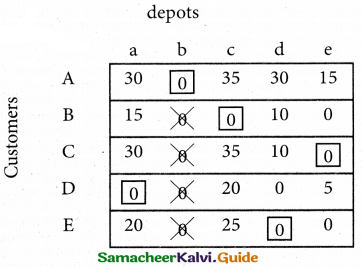Here all the five assignments have been made. The optimal assignment schedule and total distance is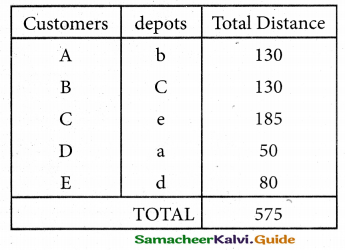∴ The optimum Distance (minimum) 575 kmsQuestion 6.
A natural truck – rental service has a surplus of one truck in each of the cities 1, 2, 3, 4, 5 and 6 and a deficit of one truck in each of the cities 7, 8, 9, 10, 11 and 12. The distance (in kilometers) between the cities with a surplus and the cities with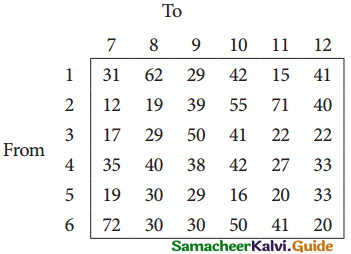How should the truck be dispersed so as to minimize the total distance travelled?
Solution:
Here the number of rows and columns are equal.
∴ The given assignment problem is balanced.
Step 1.
Select the smallest element in each row and subtract this from all the elements in its row.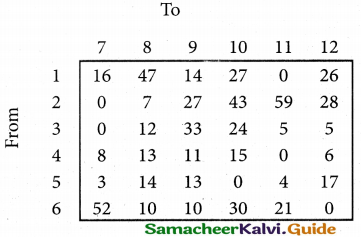Step 2.
Select the smallest element in each column and subtract this from all the elements in its column.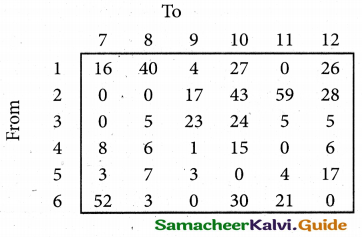Step 3.
Examine the rows with exactly one zero, mark the zero by □ mark other zeros, in its column by X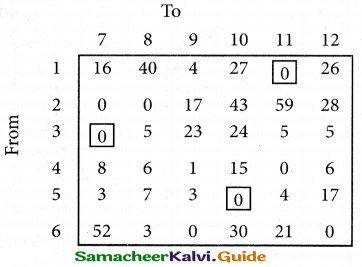Step 4.
Examine the Columns with exactly one zero. If there is exactly one zero, mark that zero by □ mark other zeros in its rows by X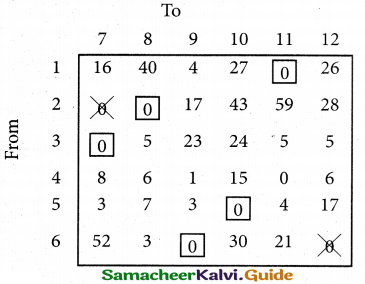Step 5.
Cover all the zeros of table 4 with five lines. Since three assignments were made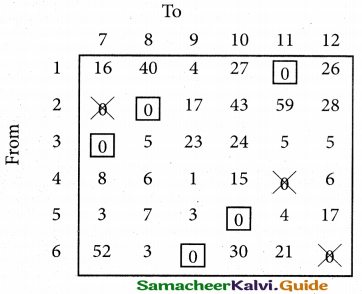Step 6.
Develop the new revised tableau. Examine those elements that are not covered by a line in Table 5. Take the smallest element. This is l(one) in our case. By subtracting 1 from the uncovered cells.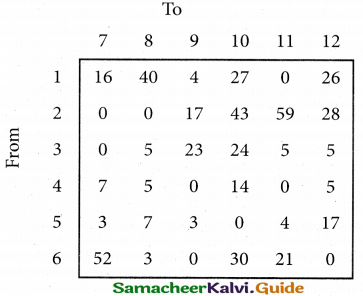Step 7.
Go to step 3 and repeat the procedure until you arrive at an optimal assignments.

Step 8.
Determine an assignment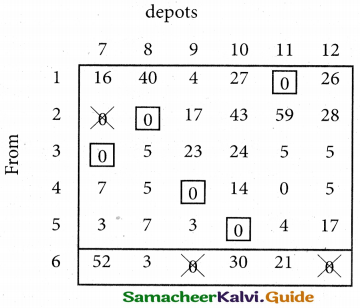Here all the six assignments have been made. The optimal assignment schedule and total distance is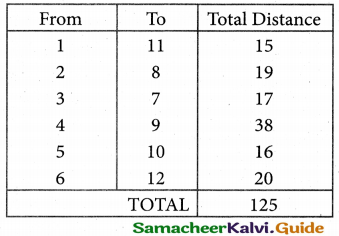∴The optimum Distance (minimum) = 125 kmsQuestion 7.
A person wants to invest in one of three alternative investment plans: Stock, Bonds and Debentures. It is assumed that the person wishes to invest all of the funds in a plan. The pay – off matrix based on three potential economic conditions is given in the following table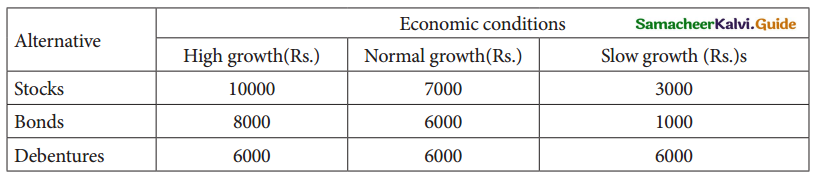Solution: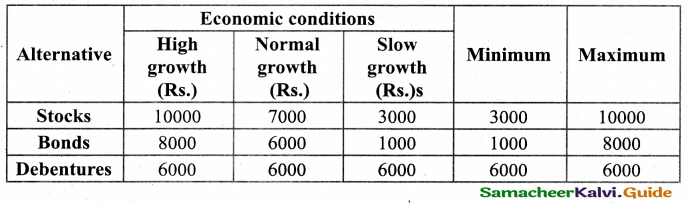(i) Maximin
Max (3000, 1000, 6000) = 6000. Since the maximum pay of is 6000, the alternative ‘Debentures’, is selected.

(ii) Minimax
Min (10000, 8000, 6000) = 6000, Since the minimum pay-off is 6000. the alternative ‘Debentures’ is selected.